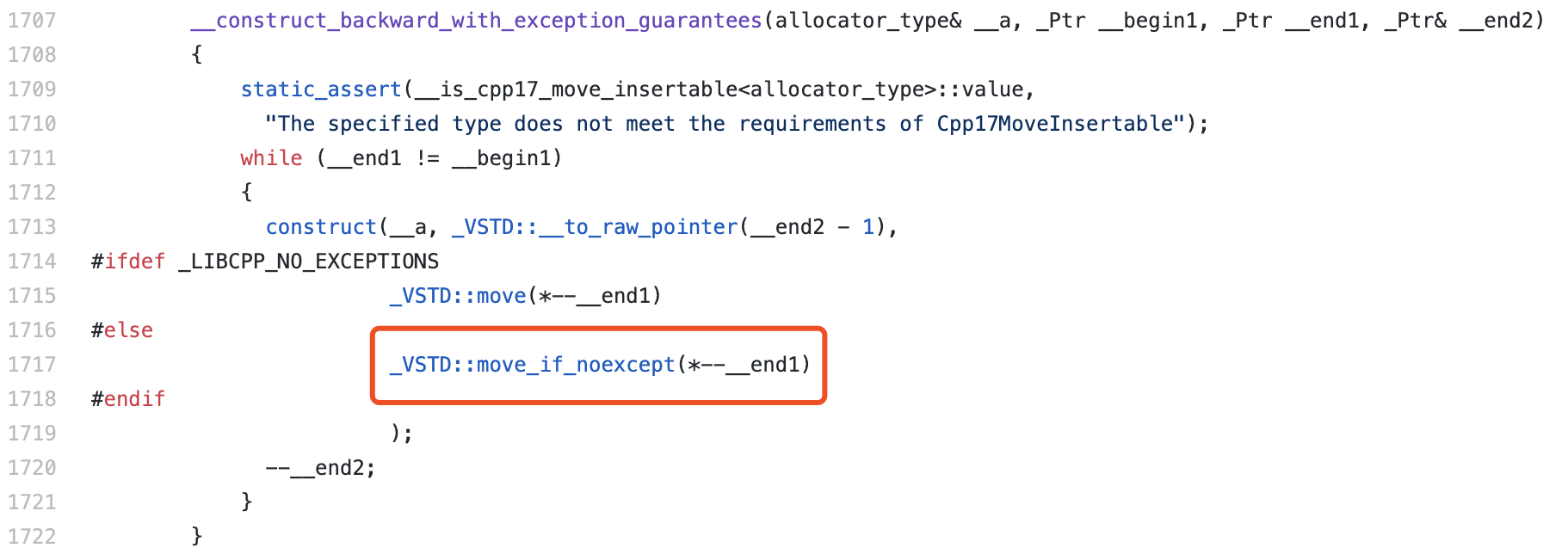X

# C++ 中的移动构造与 noexcept

“noexcept”，形如其名，使用它修饰的函数被标记为不会在执行过程中抛出异常。通常我们可以用这两种不同的方式来使用这个关键字。第一种方式，我们可以简单地将 “noexcept” 关键字放在函数声明的后面，这样该函数便会被标记为不会抛出异常。如下代码所示。

``````void excpt_func() noexcept;
``````

``````constexpr bool suppressExcept = true;
void excpt_func() noexcept (suppressExcept);
````````````MyClass(MyClass &&res) noexcept;
``````

``````#include <iostream>
#include <type_traits>

using namespace std;

struct ClassA {
int n;
ClassA(ClassA&&) = default;
};
struct ClassB {
int n;
// 标记为可能抛出异常；
ClassB(ClassB&&) noexcept(false) {};
};

int main() {
cout << boolalpha
<< is_move_constructible<ClassA>::value << endl
<< is_trivially_move_constructible<ClassA>::value << endl
<< is_nothrow_move_constructible<ClassA>::value << endl
<< is_move_constructible<ClassB>::value << endl
<< is_trivially_move_constructible<ClassB>::value << endl
<< is_nothrow_move_constructible<ClassB>::value << endl;
}
``````